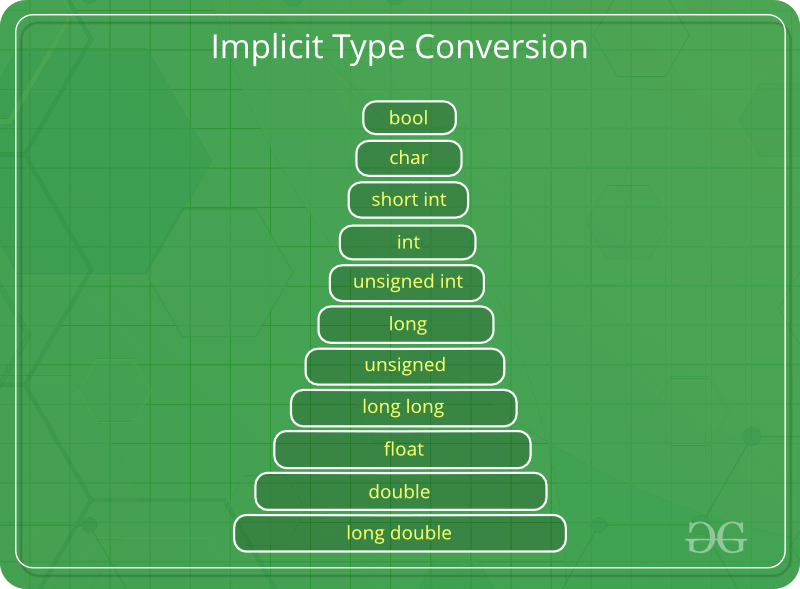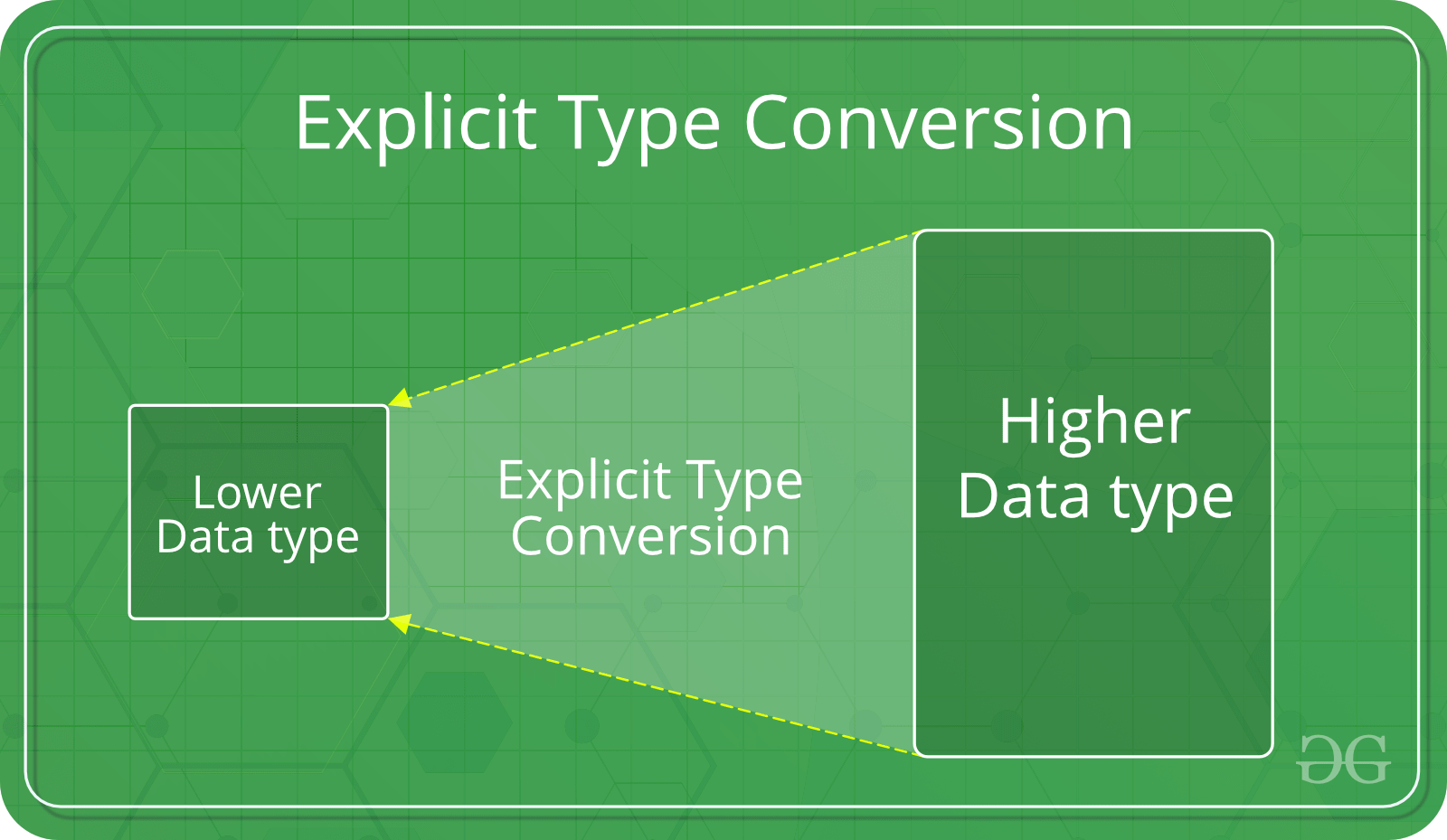Open in App
Not now

# Type Conversion in C

• Difficulty Level : Easy
• Last Updated : 25 Oct, 2022

Type conversion in C is the process of converting one data type to another. The type conversion is only performed to those data types where conversion is possible. Type conversion is performed by a compiler. In type conversion, the destination data type can’t be smaller than the source data type. Type conversion is done at compile time and it is also called widening conversion because the destination data type can’t be smaller than the source data type. There are two types of Conversion:

## 1. Implicit Type ConversionAlso known as ‘automatic type conversion’.

A. Done by the compiler on its own, without any external trigger from the user.

B. Generally takes place when in an expression more than one data type is present. In such conditions type conversion (type promotion) takes place to avoid loss of data.

C. All the data types of the variables are upgraded to the data type of the variable with the largest data type.

```bool -> char -> short int -> int ->
unsigned int -> long -> unsigned ->
long long -> float -> double -> long double```

D. It is possible for implicit conversions to lose information, signs can be lost (when signed is implicitly converted to unsigned), and overflow can occur (when long long is implicitly converted to float).

### Occurrences of Implicit Type Conversion in C

Implicit type conversion is also called automatic type conversion. Some of its few occurrences are mentioned below:

• Conversion Rank
• Conversions in Assignment Expressions
• Conversion in other Binary Expressions
• Promotion
• Demotion

Example of Type Implicit Conversion

## C

 `// An example of implicit conversion``#include ``int` `main()``{``    ``int` `x = 10; ``// integer x``    ``char` `y = ``'a'``; ``// character c`` ` `    ``// y implicitly converted to int. ASCII``    ``// value of 'a' is 97``    ``x = x + y;`` ` `    ``// x is implicitly converted to float``    ``float` `z = x + 1.0;`` ` `    ``printf``(``"x = %d, z = %f"``, x, z);``    ``return` `0;``}`

Output

`x = 107, z = 108.000000`

## 2. Explicit Type ConversionThis process is also called type casting and it is user-defined. Here the user can typecast the result to make it of a particular data type. The syntax in C Programming:

`(type) expression`

Type indicated the data type to which the final result is converted.

## C

 `// C program to demonstrate explicit type casting``#include``   ` `int` `main()``{``    ``double` `x = 1.2;``   ` `    ``// Explicit conversion from double to int``    ``int` `sum = (``int``)x + 1;``   ` `    ``printf``(``"sum = %d"``, sum);``   ` `    ``return` `0;``}`

Output

`sum = 2`

• This is done to take advantage of certain features of type hierarchies or type representations.
• It helps us to compute expressions containing variables of different data types.
• The accuracy of our result increases with the help of type conversion.
• Before performing operations, we can refer to the conversion rank hierarchy to get better results.

Must Read – Integer Promotions in C

This article is contributed by Ankita Dutta. If you like GeeksforGeeks and would like to contribute, you can also write an article on write.geeksforgeeks.org. See your article appearing on the GeeksforGeeks main page and help other Geeks.